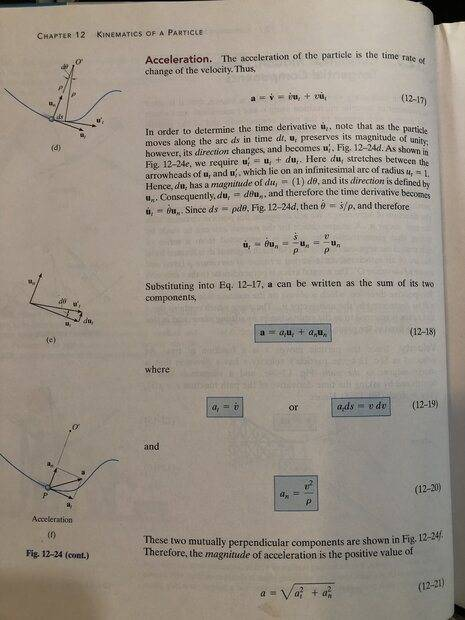# Calculations for swinging on a rope out over a lake (max. tension, final angle, etc.)

• simphys
If by the index in the ##a_t## you mean tangent to the trajectory, then I think you don't need to correct. By definition, the tangential component of the acceleration is equal to the derivative in respect to time of the magnitude of velocity. There is no velocity perpendicular to the trajectory. "Radial velocity" may reffer to diection along a radius which is not normal to the trajectory in the point of interest. Like for eliptical motion. But here too the change in the magnitude of velocity is given by the component of the force which is tangent to the trajectory and produces a tangential acceleration.
Yes.

## a_t ## is the tangential component of the acceleration. We were working in ##n-t## components.

It's correct in the special case of circular motion when only conservative forces act on the mass undergoing it.
The definition of the tangential acceleration is not related to a special trajectory or a special type of force.
Nevermind. I did not pay sufficient attention to the formula in discussion. It is not about integrating the tangential acceleration.
Sorry for the confusion.

I'm not sure I understand what needs to be done for it to be corrected.
In vectors it can be written correctly as ##\vec v.d\vec v=\vec v.\vec a.dt=\vec a.\vec v.dt=\vec a.d\vec s##.
Converting to scalars, the RHS becomes ##a_t.ds##, but I can't find a neat way to write the LHS.

Edit, .. but it could be written ##v.dv##, if ##dv## is defined as the component of the change in velocity in the direction of the existing velocity. I.e. the change in speed, treating speed as a scalar, not a magnitude.

Last edited:
Yes.

## a_t ## is the tangential component of the acceleration.
Sorry, my mistake. What I said is true but does not apply to your equation under discussion. I though that by "kinematic equation" is ment ##dv= a_t dt##.

Sorry, my mistake. What I said is true but does not apply to your equation under discussion. I though that by "kinematic equation" is ment ##dv= a_t dt##.
It's getting worse! Head is spinning! Is it not only the tangential component of the acceleration that contributes to the change in magnitude of the velocity in the ## n-t## coordinates?

Am I mis-interpreting the author's (R.C. Hibbeler) derivation here?Notice ##(12-19)##

Last edited:
It's getting worse! Head is spinning! Is it not only the tangential component of the acceleration that contributes to the change in magnitude of the velocity in the ## n-t## coordinates?
Yes, it is. This is so for any motion. But you have something else in what you call kinematic equation.

Yes, it is. This is so for any motion. But you have something else in what you call kinematic equation.

I have eq ##(12-19)## in the above derivation. It represents the magnitude of ##\boldsymbol{a_t}##.

Sorry, my mistake. What I said is true but does not apply to your equation under discussion. I thought that by "kinematic equation" is meant ##dv= a_t dt##.

From what you said above it follows from a simple application of the chain rule:

$$a_t = \frac{dv}{dt} = \frac{dv}{ds} \frac{ds}{dt} = \frac{dv}{ds} v \implies a_t \, ds = v \, dv$$

Why/Where is this supposedly inapplicable, can someone give a simple example?

Last edited:
I have eq ##(12-19)## in the above derivation. It represents the magnitude of ##\boldsymbol{a_t}##.

From what you said above it follows from a simple application of the chain rule:

$$a_t = \frac{dv}{dt} = \frac{dv}{ds} \frac{ds}{dt} = \frac{dv}{ds} v \implies a_t \, ds = v \, dv$$

Why/Where is this supposedly inapplicable, can someone give a simple example?
I think it hinges on how dv is being defined. See edit in post #38.